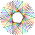CipherFeb 3, 2017

NASA Makes an EPIC Update on 33rd day of the year

NASA's post -

This is by far my favorite story that came out on the 33rd day of this year, I knew NASA was gonna give us something fun
This is all very funny, EPIC = 33, and here we have update about images from EPIC camera on 33rd day of the year, and the first picture they display was taken seven days before this it was released
EPIC = 5+16+9+3 = 33 (Ordinal)
EPIC = 31+42+35+29 = 137 (Francis Bacon) - 33rd prime number is 137
seven days = 1+5+4+5+5 + 4+1+7+1 = 33 (Reduced)
Thrity three = 2+8+9+9+2+7 + 2+8+9+5+5 = 66 (Reduced)
February Second = 6+5+2+9+3+1+9+7 + 1+5+3+6+5+4 = 66 (Reduced)
The first sentence under quotation marks is interesting as well
"The 'enhanced' color images make land features more visible," = 2+8+5 + 5+5+8+1+5+3+5+4 + 3+6+3+6+9 + 9+4+1+7+5+1 + 4+1+2+5 + 3+1+5+4 + 6+5+1+2+3+9+5+1 + 4+6+9+5 + 4+9+1+9+2+3+5 = 219 (Reduced)

EPIC camera was 'launched' aboard DSCOVR spacecraft
Launch site - Capre Canaveral ... CC - 33
It's funny that the 'satellite' was launched by Falcon 9v1.1, I have a post on Falcon tribute from SpaceX to 911 - SpaceX-ElonMusk-911-tribute

DSCOVR was launched on 2/11/2015
EPIC camera = 5+7+9+3 + 3+1+4+5+9+1 = 47 (Reduced)
And in this post we have "Sasha Marshak" (DSCOVR deputy project scientist) talking about EPIC update
Sasha Marshak = 100+1+100+8+1 + 40+1+90+100+8+1+20 = 470 (English)

Her full name gematria is reflection of 156th prime number and it also corresponds to the date of release
February Second = 6+5+2+18+21+1+18+25 + 19+5+3+15+14+4 = 156 (Ordinal)
Sasha Marshak = 19+1+19+8+1 + 13+1+18+19+8+1+11 = 119 (Ordinal)
EPIC camera = 5+70+9+3 + 3+1+40+5+90+1 = 227 (English)
119+191+911 = 1221
227+272+722 = 1221

The news from NASA comes 722 days after the spacecraft was 'launched'. It's a reflection of 227 (Twenty two divided by seven = 3.14 (Pi), NASA likes to code Pi and numbers related to Golden ratio - for example 137.5° is known as Golden angle and it is seen in the geometry of plants, 33rd prime number is 137)
DSCOVR was launched on - "February Eleventh" = 6+5+2+9+3+1+9+7 + 5+3+5+4+5+5+2+8 = 79 (Reduced) and the news comes on 2/2 (22nd prime number is 79)
"EPIC is a four megapixel CCD camera"
four megapixel CCD camera = 6+50+200+80 + 30+5+7+1+60+9+300+5+20 + 3+3+4 + 3+1+30+5+80+1 = 903 (Jewish)
It was launched by Falcon 9 rocket
Falcon Nine = 6+1+12+3+15+14 + 14+9+14+5 = 93 (Ordinal)
The launch was on 42nd day of the year (42nd triangular number is 903) - the satellite was launched in only month with 42 gematria
February = 6+5+2+9+3+1+9+7 = 42 (Reduced)

The date of launch and 2/2/2017 have matching date numerology of 21
2/11/2015 = 2+11+2+0+1+5 = 21
2/2/17 = 2+2+17 = 21
Saturn = 1+1+2+3+9+5 = 21 (Reduced)
Saturn = 19+1+20+21+18+14 = 93 (Ordinal)
Twenty one = 2+5+5+5+2+7 + 6+5+5 = 42 (Reduced)
Magic Cube of Saturn connects to number 42 (sum of all rows, columns and 3-D diagonals in 3x3x3 Cube)

They always make sure to drop seemingly random numbers in their posts - "NOAA's DSCOVR satellite that takes 10 narrow-band spectral images of the entire sunlit face of Earth from 317 to 780 nanometers."

317 and 780 are both interesting, 780 is 39th triangular number, there's 39 books of the Old Testament that the Jews go by, also reflection is the number of Saturn (God of the Old Testament)
This goes well with the date of release being 33rd day
February Second = 6+5+2+9+3+1+9+7 + 1+5+3+6+5+4 = 66 (Reduced)
Thirty three = 2+8+9+9+2+7 + 2+8+9+5+5 = 66 (Reduced)
It might also be corresponding to date numerology
Sixty six = 1+9+6+2+7 + 1+9+6 = 41 (Reduced) - 13th prime number connected to Saturn very much as well
2/2/2017 = 2+2+20+17 = 41

"EPIC takes a new picture approximately every hour from mid-April to mid-October", which means they are gonna be 'taking pictures' for six months
six months = 1+9+6 + 4+6+5+2+8+1 = 42 (Reduced)
mid-October = 30+9+4+50+3+100+50+2+5+80 = 333 (Jewish)
It's funny that mid-october has 333 gematria since October is the only month with 33 gematria
October = 6+3+2+6+2+5+9 = 33 (Reduced)
There was interesting unofficial name this DSCOVR satellite had
GoreSat = 7+50+80+5+90+1+100 = 333 (Jewish)
GoreSat = 33+15+18+5+45+1+20 = 137 (Francis Bacon) - 33rd prime
Triana = 2+9+9+1+5+1 = 27 (Reduced)
Triana = 100+80+9+1+40+1 = 231 (Jewish)
Triana = 200+90+9+1+50+1 = 351 (English)

They always pick the names very carefully, 153 and 351 are special numbers connected to God, 17th triangular number is 153 and 26th is 351 (God = 17/26)

The writer of this post is Karl Hille and for the rest of this post he talks about the new features on their bullshit website
Karl Hille = 20+1+90+30 + 8+9+30+30+5 = 223 (English)
The synagogue of Satan = 223, also it is reflection of the number of Skull & Bones

1.The coding on anything NASA is completely insane, who do these jokers think they're kidding? It's hard to believe anything nowadays when the parallels are bigger than the galaxy itself.

1.NASA is Saturn worship at the highest level haha, it has become quite funny to me

2.780 - 317 = 463, 463 is the 90th prime, Ninety = 33

Always do the most basic equations!!!

1.Damn, I always forget it :D